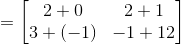## Example Questions

### Example Question #1 : How To Add Matrices

Simplify: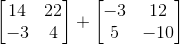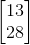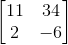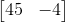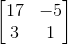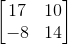Explanation:

Matrix addition is very easy! All that you need to do is add each correlative member to each other. Think of it like this: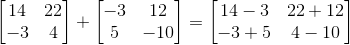Now, just simplify:### Example Question #12 : Sum Or Difference Of Two Matrices

Simplify: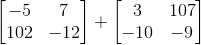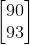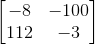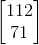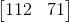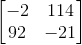Explanation:

Matrix addition is really easy—don't overthink it! All you need to do is combine the two matrices in a one-to-one manner for each index: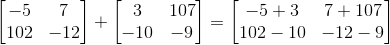Then, just simplify all of those simple additions and subtractions: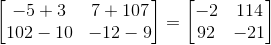### Example Question #3 : How To Add Matrices

What is the value of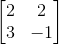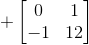?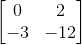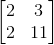You cannot add these two matrices because their indices do not line up.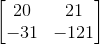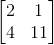Explanation:

To add matrices you simply add the numbers in the same position as each other.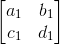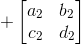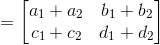Plugging the given values into the above formula, we are able to solve the question.